# Introduction

• Made available UCL Week 10: 2 November 2021
• Due UCL Week 12: 18 November 2021, 13:00 London Time.
• This ICA consists of five questions, worth 50 points;
• another 10 points will be given based on presentation, so that the total available points is 60.
• This ICA is worth 30 percent of your grade for this module.
• Many questions will require you to write and run R/Python code.
• The ICA must be completed in R Markdown/Juptyer Notebook and typeset using Markdown with Latex code, just like the way our module content is generated. You can choose to use either R or Python.
• Please hand in the html file and the Rmd/ipynb source file.
• As usual, part of the grading will depend on the clarity and presentation of your solutions.
• You are to do this assignment by yourselves, without any help from others.
• You are allowed to use any materials and code that was presented so far.
• Do not search the internet for answers to the ICA.
• Do not use any fancy code or packages imported from elsewhere.

## Plagiarism and collusion

Please familarize yourself with the following excerpt on plagiarism and collusion from the student handbook

By ticking the submission declaration box in Moodle you are agreeing to the following declaration:

Declaration: I am aware of the UCL Statistical Science Department’s regulations on plagiarism for assessed coursework. I have read the guidelines in the student handbook and understand what constitutes plagiarism. I hereby affirm that the work I am submitting for this in-course assessment is entirely my own.

# Questions

## 1.) Empirical distribution [10 points]

• Let $$X_1, \ldots, X_n$$ be i.i.d. random variables all with cdf $$F$$. Show that for every $$x \in \mathbb{R}$$, we have

$\frac{1}{n} \sum_{i=1}^n \mathbf{1}[ X_i \leq x] \to F(x). \ \text{[5 points]}$

• Demonstrate this fact by running simulations of a large number of random variables that are uniformly distributed in the unit interval $$[0,1]$$. [5 points]

## 2.) Generating random variables [10 points]

• Show that if $$X$$ is a continuous random variable taking values on $$D$$ with a cdf that is strictly increasing on $$D$$, then the random variable $$F(X)$$ is uniformly distributed on the unit interval $$[0,1]$$. [3 points]

• Show that if $$U$$ is uniformly distributed in $$[0, \tfrac{\pi}{2}]$$, then $$\sin^2(U)$$ has the beta distribution with parameters $$(\tfrac{1}{2}, \tfrac{1}{2})$$. [3 points]

• Suppose that you have access to a true source of randomization given by say radioactive decay; that is, you have access to independent random variables that are exponentially distributed with rate $$1$$. Show that you can generate random variables with the beta distribution with parameters $$(\tfrac{1}{2}, \tfrac{1}{2})$$. [2 points]

• Demonstrate your procedure in the last question, by computer simulations, and plot a histogram of the results against pdf of the beta $$(\tfrac{1}{2}, \tfrac{1}{2})$$. [2 points]

## 3.) Random walk [5 points]

Let $$S_n = X_1 + \cdots + X_n$$, where $$X_i$$ are i.i.d. random variables, with $\mathbb{P}(X_1 = 1) = \tfrac{1}{2} = \mathbb{P}(X_1 = -1).$

Let $L_n = \# \{ 1 \leq k \leq n : S_k >0\}.$ Demonstrate, by simulations, that $$L_n/n$$ converges in distribution to the beta $$(\tfrac{1}{2}, \tfrac{1}{2})$$ distribution.

## 4.) Exporting and importing data [5 points]

• Simulate $$250$$ random variables that are uniformly distributed in $$[0,1]$$.
• Export them to a tab delimited text file named export.txt.
• Now import them back and save them under the variable imported.
• Plot a probability histogram of the imported data. You may need to do some processing as the values may be in a table, rather than a vector.

## 5.) Estimating the stationary distribution [10 points]

• Suppose you are given the output of a $$100000$$ steps of a irreducible and aperiodic finite state Markov chain. Carefully explain how you could estimate the stationary distribution for this Markov chain, and why you estimator is reasonable. [5 points]

• Import the data from the file markovchain.txt and use this data and your method above to estimate the stationary distribution. [5 points]

## 6.) Poisson processes [10 points]

Suppose a shop that operates daily in the time interval $$[a,b]$$. It has customers arriving according to a Poisson process of intensity $$3$$ in the time interval $$[a, c)$$, and a Poisson process of intensity $$5$$ in the time interval $$[c,b)$$; here $$a$$ and $$b$$ are known, but $$c$$ is unknown. You can imagine the shop keeper notices that at some point in the day, the shop seems to get busier. The shop keeper has a log of all the arrival times, for each of $$n$$ days of operation, where $$n$$ is large.

• Given an open interval $$(r,s) \subset [a,b]$$, explain how you can use the shop keeper’s log to make a good guess at whether or not $$(r,s)$$ contains the unknown time $$c$$; show that as $$n \to \infty$$ you will know with certainty whether $$c \in (r,s)$$. Carefully explain your answer. [5 points]

• Demonstrate your answer by running simulations; for example, choose $$a=0$$, $$b=8$$, and $$c=4$$, and simulate the arrivals to generate the shop keeper’s log. Now apply your method with the intervals $$(2.7, 4.3)$$ and $$(5,6)$$. [5 points]

# Solutions

## Empirical Distributions

• Fix $$x \in \mathbb{R}$$. We observe that the Bernoulli random variables $$\mathbf{1}[X_i \leq x]$$ are i.i.d. with mean $$\mathbb{P}(X_i \leq x) = F(x)$$. Thus the desired convergence is immediate from the law of large numbers.

• We will run our simulations in Python.

import numpy as np

u = [np.random.uniform() for _ in range(1000)]
t = t=np.linspace(0,1,num=1000)
def emperical(x):
return (1/len(u)) * sum(  k <= x for k in u)

import matplotlib.pyplot as plt
plt.plot(t,t, label='uniform cdf')
plt.plot(t,emperical(t), label='empirical distribution')
plt.legend(loc='upper left')
plt.show()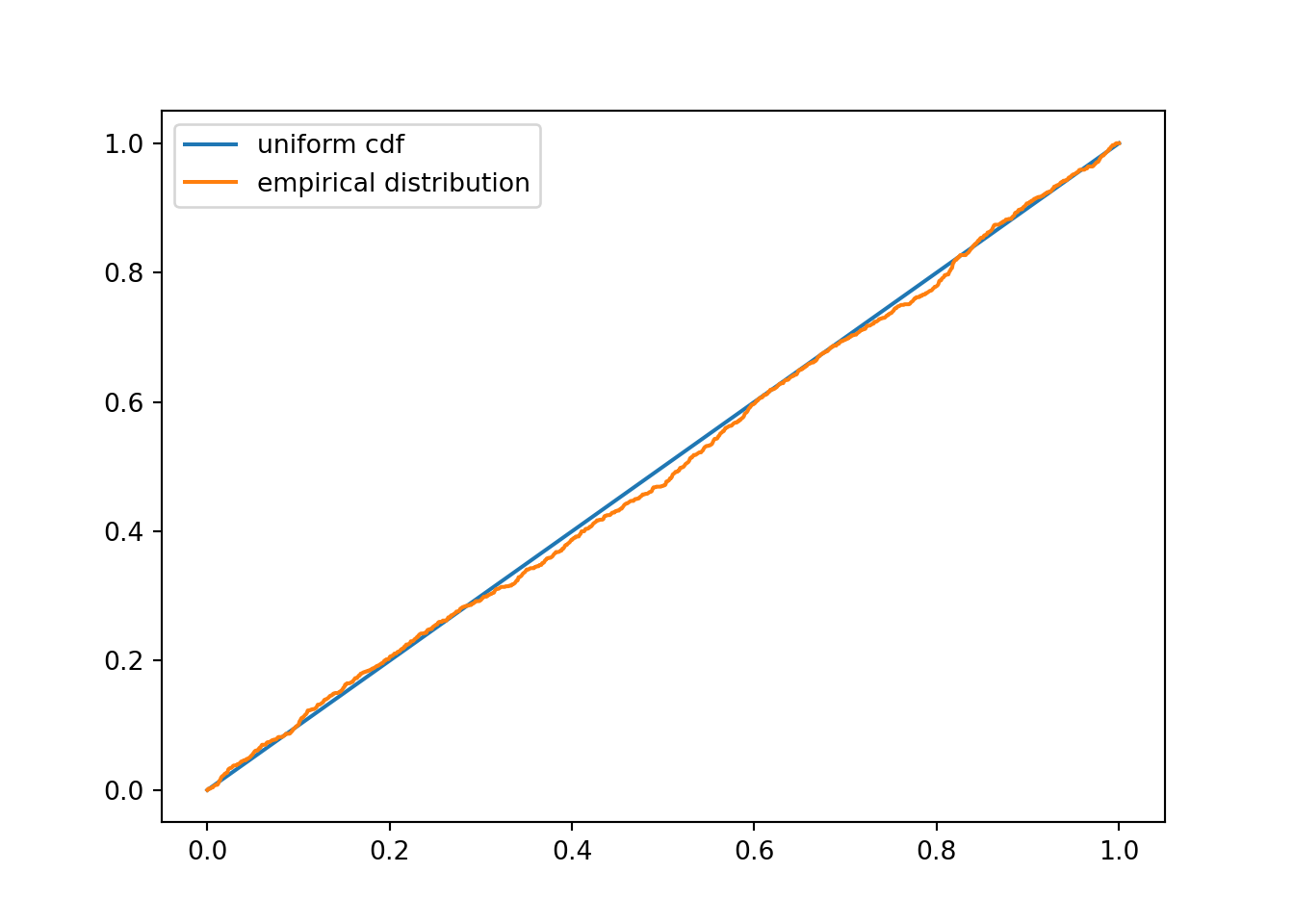## Generating random variables

• Note that $$F(X)$$ takes values in $$[0,1]$$. Let $$x \in [0,1]$$, then $$\{ F(X) \leq x \} = \{ X \leq F^{-1}(x)\}$$. Thus $\mathbb{P}(F(X) \leq x ) = \mathbb{P}(X \leq F^{-}(x)) = F( F^{-1}(x)) = x,$
as desired.

• Note that $$\sin^2(U)$$ takes values in $$[0,1]$$. For $$x \in [0,1]$$, we have $\mathbb{P}(\sin^2(U) \leq x) =\mathbb{P}( U \leq \sin^{-1}(\sqrt{x}) = \frac{2}{\pi} \cdot \sin^{-1}(\sqrt{x});$ hence taking a derviative with respect to $$x$$, we obtain the pdf $x \mapsto \frac{1}{\pi \sqrt{x(1-x)}},$ as desired.

• If $$X$$ is exponential, then we know that cdf is given by $F(x) = 1 - e^{-x}.$ Thus the first part gives that $$F(X)$$ is uniformly distributed on $$[0, 1]$$, so that $$\tfrac{\pi}{2} F(X)$$ is uniformly distributed on $$[0, \tfrac{\pi}{2}]$$. Now the previous part gives that $$\sin^2(\tfrac{\pi}{2} F(X))$$ has the required beta distribution.

• We do our simulations in Python

def F(x):
return 1-np.exp(-x)

print(1+1)
## 2
x = np.random.exponential(size=10000)

trans = (np.sin((np.pi/2)*F(x)))**2

suppress=plt.hist(trans, bins = 25, density=True, label='Proability Histogram')
t=np.linspace(0.001,0.999,num=100)
plt.plot(t, 1/ (np.pi * np.sqrt(t*(1-t))), label='Exact Density')
plt.legend(loc='upper left')
plt.show()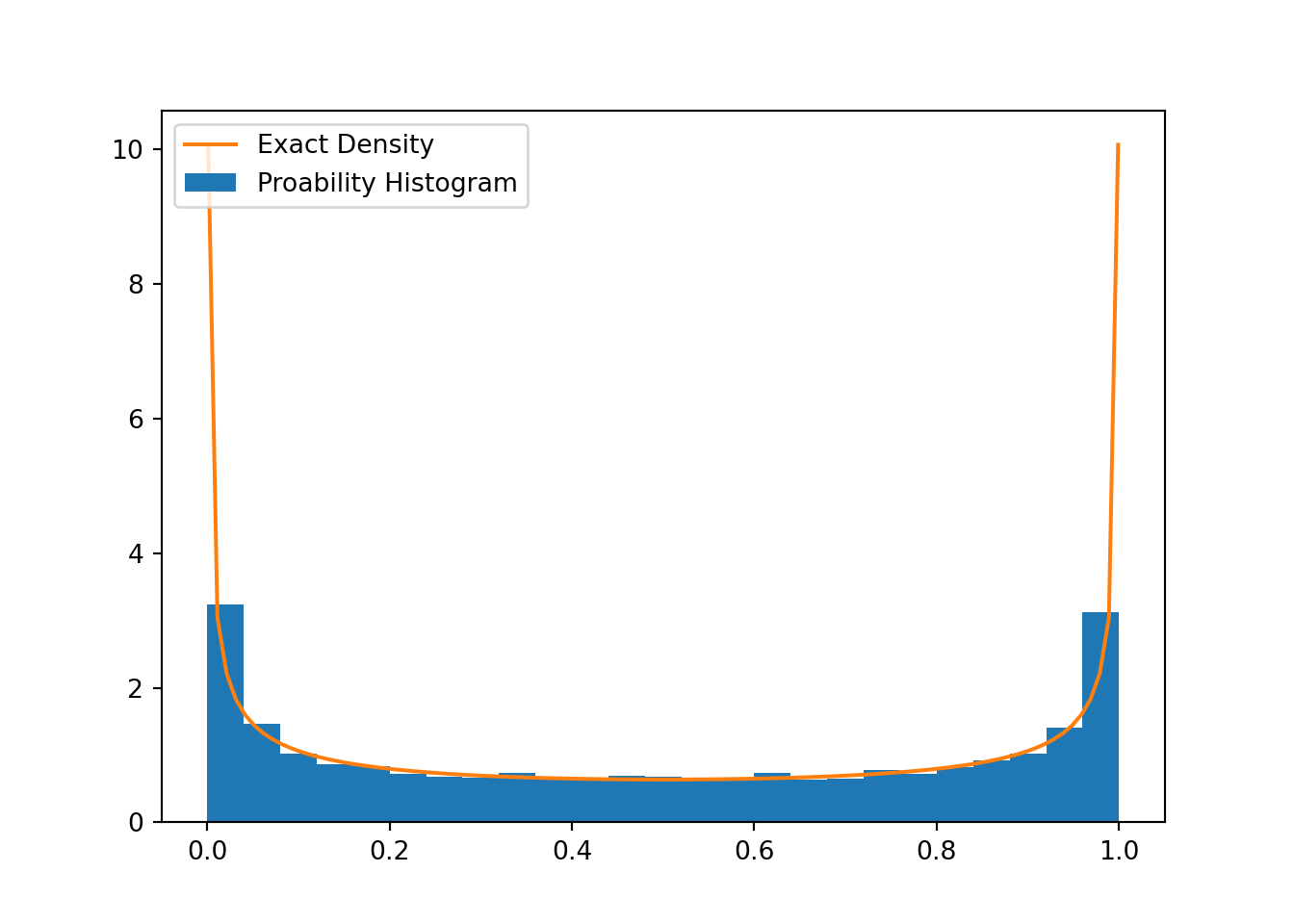## Random walk

We do our simulations in Python

def L(n):
s=np.array()
for i in range(n):
x= 2*np.random.binomial(1,0.5,1)-1
s = np.append(s, s[-1]+x)
return sum(0<k for  k in s)

LL = [L(5000)/5000 for _ in range(500)]

suppress=plt.hist(LL, bins = 25, density=True, label='Proability Histogram')
t=np.linspace(0.001,0.999,num=100)
plt.plot(t, 1/ (np.pi * np.sqrt(t*(1-t))), label='Exact Density')
plt.legend(loc='upper left')
plt.show()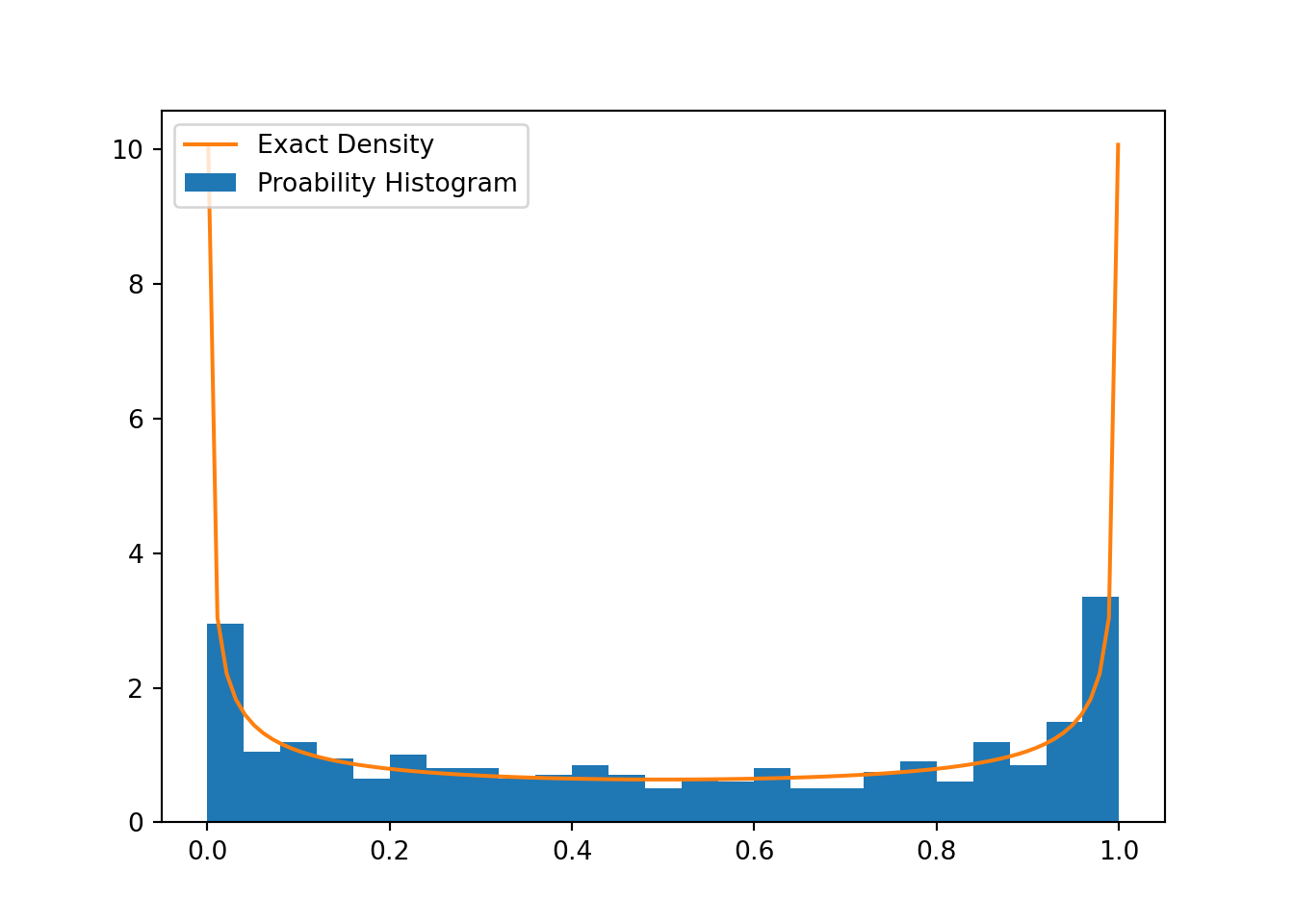## Exporting and importing data

We will do this exercise in R.

x = runif(250)
write.table(x, "export.txt", sep=",")
z= imported[,]
hist(z, prob=TRUE)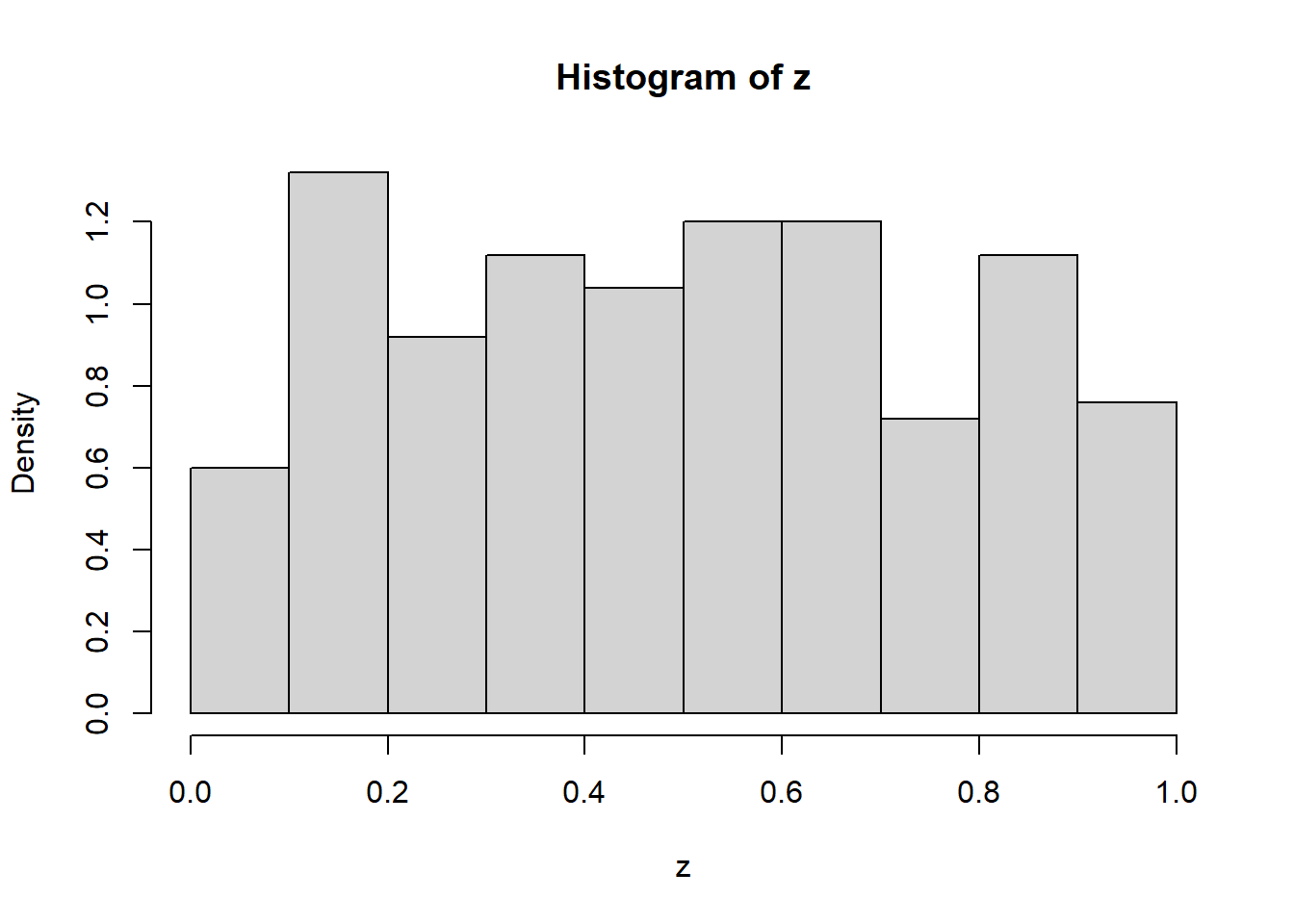## Estimating the stationary distribution

• Since the chain is on a finite state space and irreducible and aperiodic, it has a unique stationary distribution $$\pi$$, and we know that a version of the law of large numbers gives that for every state $$s$$, we have

$\frac{1}{n} \sum_{i=1}^n \mathbf{1}[X_i = s] \to \pi(s).$ Thus for each state, we simply need to count the number of occurrences and divide by $$100000$$, to get its approximate probability under $$\pi$$.

• A quick scan of the file shows there are $$4$$ states: $$1,2,3,4$$. With R, we have:
z =read.table("markovchain.txt", sep=",")
z= z[,]

b1 = seq(-1,5, by=1)
hist(z, prob=TRUE, breaks=b1)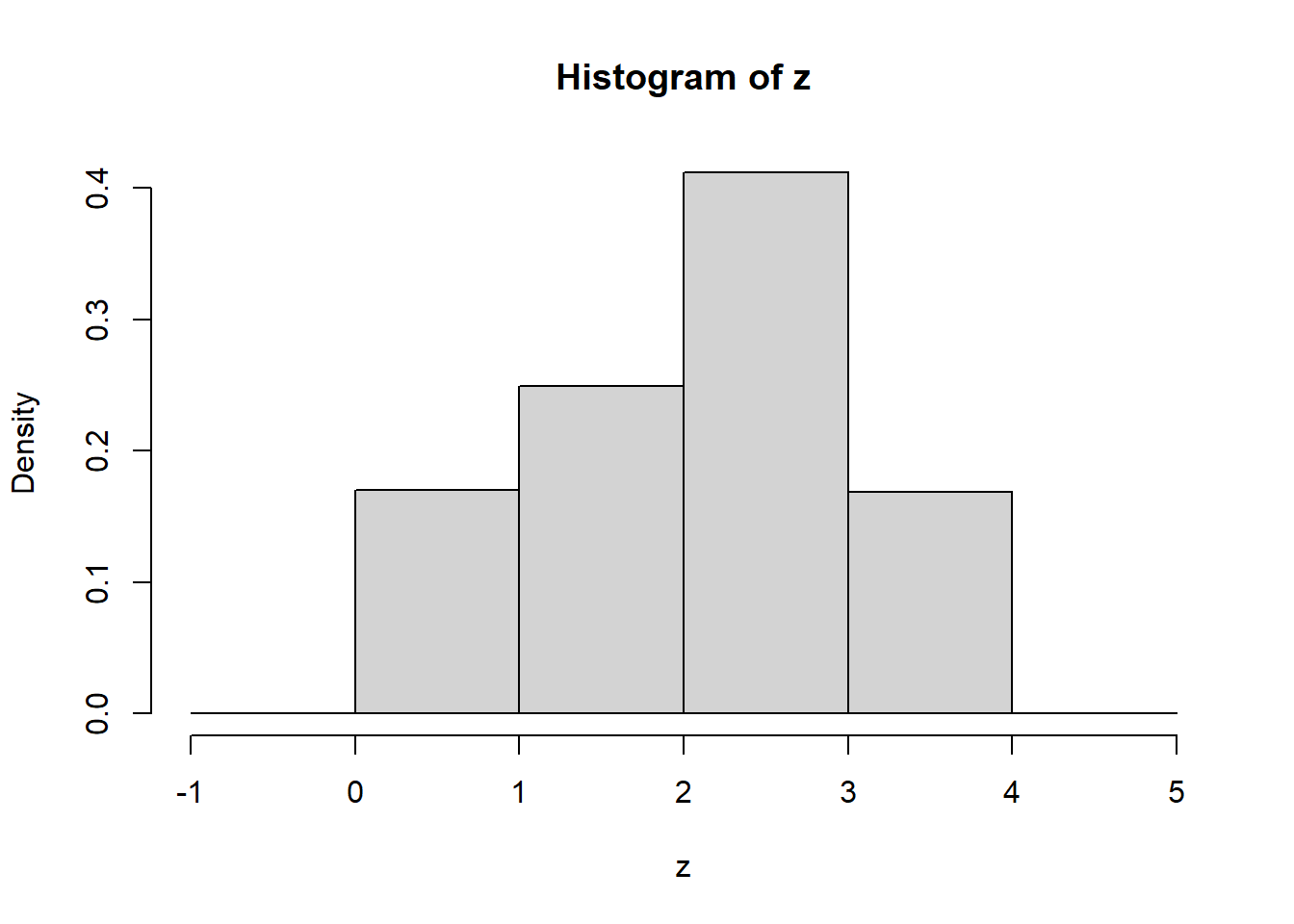sum(z==1)/100000
##  0.17023
sum(z==2)/100000
##  0.24957
sum(z==3)/100000
##  0.41172
sum(z==4)/100000
##  0.16849

## Poisson processes

• First, suppose that $$(r,s)$$ does not contain the point $$c$$, then the Poisson process restricted to the time interval $$[r,s]$$ has either intensity $$3$$ or intensity $$5$$, for the whole time interval $$(r,s)$$. If we let $$X_i$$ be the number of arrivals in the time interval $$(r,s)$$ for each log entry $$i$$, then we know that $$X_i$$ is a Poisson random variable with mean $$\lambda(s-r)$$, where $$\lambda$$ is either $$3$$ or $$5$$. Assuming the log entries are independent, then the law of large numbers gives that

$\frac{1}{n} \sum_{i=1} ^n X_i \to \mathbb{E} X_1 = \lambda(s-r);$

Similarly, if $$(r,s)$$ does contain the point $$c$$, then it is not difficult to argue that $$X_i$$ has mean $$3(c-r) + 5(s-c)$$, and the law of large numbers again will detect this; in particular, we have the convergence

$\frac{1}{(s-r)n} \sum_{i=1} ^n X_i \to \lambda;$

if $$\lambda \in (3,5)$$, then the interval contains $$c$$, and if $$\lambda \in \{3,5\}$$, it does not contain $$c$$.

• We do our simulations in R. We expect the first
arr <- function(end,intensity){
T = rexp(1,intensity)
while(T[length(T)] < end){
T <- c(T,  (T[length(T)] + rexp(1,intensity))  )
}
T <- T[-length(T)]   # remove the last arrival, as it will have past the end
T
}

record <- function(){
first=arr(4,3)
second=4+arr(4,5)
c(first, second)
}

counts <- function(r,s){
x = record()
sum( r < x & x< s)

}

z=replicate(10000, counts(2.7, 4.3))
mean(z)/(4.3-2.7)
##  3.360375
z=replicate(10000, counts(5, 6))
mean(z)/(6-5)
##  4.9778
• End of Solutions